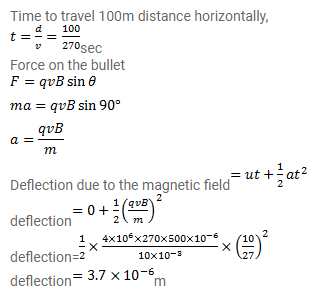# A 10g bullet having a charge of

Question:

A $10 \mathrm{~g}$ bullet having a charge of $4.00 \mu C$ is fired at a speed of $270 \mathrm{~m} / \mathrm{s}$ in a horizontal direction. A vertical magnetic field of $500 \mu T$ exists in the space. Find the deflection of the bullet due to the magnetic field as it travels through $100 \mathrm{~m}$. Make appropriate approximations.

Solution: